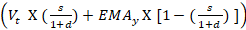# Moving Average (MA) Explained for Traders

Moving Average – Talking Points:What is a moving average?

• How do you calculate moving average?
• What is the purpose of moving averages?
• How do you interpret moving averages?

## WHAT IS A MOVING AVERAGE?

In technical analysis, the moving average is an indicator used to represent the average closing price of the market over a specified period of time. Traders often make use of moving averages as it can be a good indication of current market momentum.

The two most commonly used moving averages are the simple moving average (SMA) and the exponential moving average (EMA). The difference between these moving averages is that the simple moving average does not give any weighting to the averages in the data set whereas the exponential moving average will give more weighting to current prices.

## HOW DO YOU CALCULATE MOVING AVERAGE?

As explained above, the most common moving averages are the simple moving average (SMA) and the exponential moving average (EMA). Almost all charting packages will have a moving average as a technical indicator.

The simple moving average is simply the average of all the data points in the series divided by the number of points.

The challenge of the SMA is that all the data points will have equal weighting which may distort the true reflection of the current market’s trend.

The EMA was developed to correct this problem as it will give more weighting to the most recent prices. This makes the EMA more sensitive to the current trends in the market and is useful when determining trend direction.

Where:

A= Is each of the data points

n = Number of time periods

For example, looking at a 5-day SMA on a daily chart of EUR/USD and the closing prices over the 5 days are as follows:

Day 1: 1.321

Day 2: 1.301

Day 3: 1.325

Day 4: 1.327

Day 5: 1.326

SMA = (1.321 + 1.301 + 1.325 + 1.327 + 1.326)/5

SMA = 6.6/5

SMA = 1.32

Exponential Moving Average:

EMA =Where:

EMAt​= EMA today

Vt​= Value today

EMAt​ = EMA today

s =smoothing

d = number of days​

Steps for calculating EMA:

1. Calculate the SMA for the particular time period

2. Calculate the multiplier for weighting the EMA using the formula:

[2 ÷ (selected time period + 1)]. So, for a 10-day moving average, the multiplier would be [2/(10+1)]= 0.01818.

3. Use the smoothing factor combined with the previous EMA to arrive at the current value.

Date Of Update: 30 March 2022, 10:46